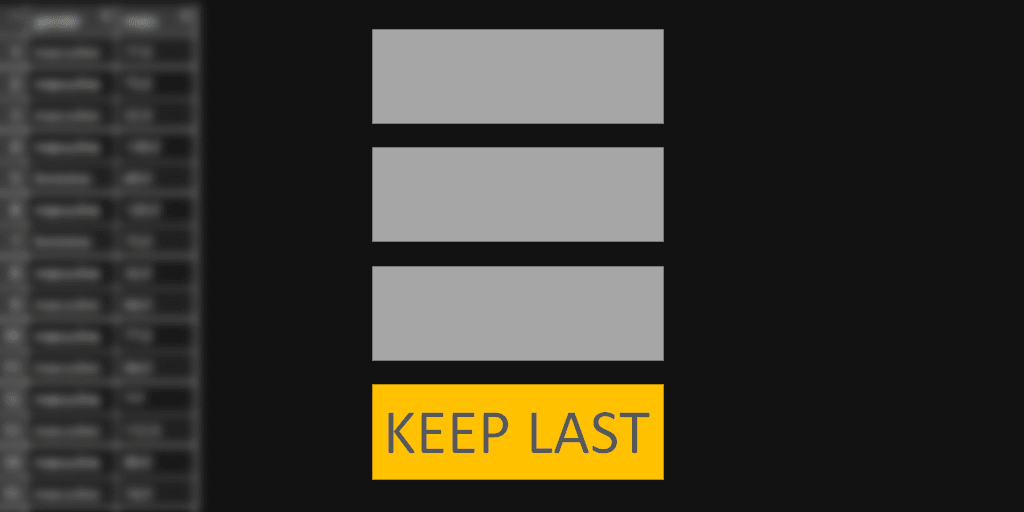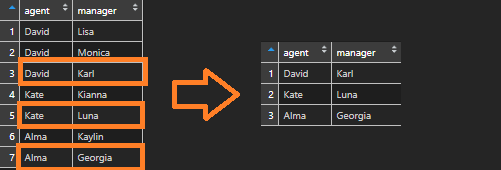# Remove duplicates and keep last in RHere is how to remove duplicates but keep the last row in the R data frame.

Here is my data frame that contains agents and managers. Let’s say the last one is that I would like to get.```df <- data.frame(
agent = as.character(c("David", "David", "David", "Kate", "Kate", "Alma", "Alma")),
manager = as.character(c("Lisa", "Monica", "Karl", "Kianna", "Luna", "Kaylin", "Georgia"))
)```

It is easy to keep the last unique record by using dplyr. Distinct functions return the first record and that is the reason you should use a little workaround.

Group by at least one category that you are interested in. Function row_count will get the count of rows by each group. Filter by last row number, which equals the result of function n. N that gives the current group size.

```df <- df %>%
group_by(agent) %>%
filter(row_number() == n())```

How to get top or bottom values by each group in R